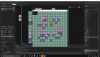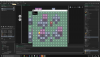# Collision Issues in 2.5D topdown platformer

#### TulioHenry

##### Member
I'm testing some features to add to my future games, and decided to try adding fake 3d platforms where all objects would have a Z axis.
Until now everything was working, i added an depth sorting system and added a better movement code to the player, but some collisions don't work so well, some objects the player can enter from a certain side, and others from the same type the collision works.

//obs: the highlighted squares are the object collision, some of them don't appear by the depth sorter. Also the objects have their z value written on them, and zfloor is the zvalue of the block i'm stepping on

This is when the collision is not working:this is when it is working://player step
GML:
``````kJump = keyboard_check_pressed(vk_space);
kUp = keyboard_check(ord("W"));
kDown = keyboard_check(ord("S"));
kLeft = keyboard_check(ord("A"));
kRight = keyboard_check(ord("D"));
kRun = keyboard_check(vk_shift);
kWalk = keyboard_check(vk_control);

//change Speed
if(kRun || kWalk){
spd = abs((kRun*runspd)-(kWalk-walkspd));
}else{
spd = normalspd;
}

moveX = 0;
moveY = 0;

var imputDirection = point_direction(0,0,kRight - kLeft,kDown - kUp);
var imputMagnitude = (kRight - kLeft != 0 || kDown - kUp != 0);
moveX = lengthdir_x(imputMagnitude * spd, imputDirection);
moveY = lengthdir_y(imputMagnitude * spd, imputDirection);

//jump on z
if(kJump){
//if on ground
if (z <= zfloor)
{
zjump = true; /*you are now in the air*/
}
}

//z jump (when space pressed)
if (zjump == true)
{
z += zspeed /*z pos goes up*/
}
//if not ontop of block
if (!instance_place(x,y,o_block_par))
{
zfloor = 0; /*zfloor is ground level*/

}
//if not on ground
if (!z <= zfloor)
{
z -= zgrav; /*apply downforce on z pos*/
zgrav += 0.2; /*grav gets stronger each step*/
}
//stop falling when you hit zfloor
if (z <= zfloor+1/*+1 for sticking glitch on ground*/)
{
z = zfloor;    /*snap z pos to on ground*/
zgrav = 0; /*stop applying downforce*/
zjump = false; /*no longer in the air*/
}

///update zfloor
if(place_meeting(x,y,o_block_par)){
instbelow = instance_place(x,y,o_block_par);
zfloor = instbelow.z
}

//colisions
if(moveX != 0){
var collisionH = instance_place(x+moveX,y,o_block_par); //instance_place para comparar o z
if(collisionH){
if(collisionH.z > z){ //if it's bigger than me
repeat(abs(moveX)){
if(!place_meeting(x+sign(moveX),y,o_block_par)){
x += sign(moveX);
}else break;
}
moveX = 0;
}

}
}

if(moveY != 0){
var collisionV = instance_place(x,y+moveY,o_block_par); //instance_place para comparar o z
if(collisionV){
if(collisionV.z > z){ //if it's bigger than me
repeat(abs(moveY)){
if(!place_meeting(x,y+sign(moveY),o_block_par)){
y += sign(moveY)
}else break;
}
moveY = 0;
}
}
}

x += moveX;
y += moveY;``````
//obs again: this issue is only happening with the 32 blocks

Last edited:

#### TheouAegis

##### Member
(!z <= zfloor)
! Is on the wrong side of the parentheses. .... And in this case you could just check >

#### TulioHenry

##### Member
! Is on the wrong side of the parentheses. .... And in this case you could just check >
I fixed that part but i'm still with the collision issue.

i don't know if i can check other collision while i'm over a collision, beacause it's very strange as only some block show this collision error and the 16 block don't show the same problem

#### TheouAegis

##### Member
Well you are using place_meeting(). You can use instance_place_list() and find the instance in the list with the highest z.

#### TulioHenry

##### Member
Well you are using place_meeting(). You can use instance_place_list() and find the instance in the list with the highest z.
Tried that now, but yet the same result.
well i suppose that i can check the other collision too, as the other sides of the block collide normally with the player, its just a specific side that it does not work